Next: Expectation and Arg Max Up: CEM - A Maximum Previous: Conditional Constraints vs. Joint

# Applying the Model

Assume we have a fully optimized conditional model of the form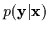from many examples of data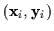through the use of a an algorithm such as CEM. Our typical scenario is to use this model and its estimated parameters to predict an output response given an input observation.

During runtime, we need to quickly generate an output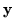given the input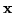. Observing an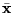value turns our conditional modelinto effectively a marginal density over(i.e.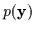). The observedmakes the gates act merely as constants, Gm, instead of as Gaussian functions. In addition, the conditional Gaussians which were original experts become ordinary Gaussians when we observeand the regressor term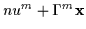becomes a simple mean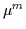. If we had a conditioned mixture of M Gaussians, the marginal density that results is an ordinary sum of M Gaussians in the space ofas in Equation 7.33.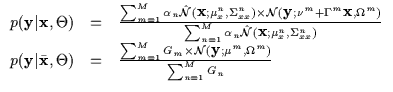(7.33)

Observe the 1D distribution in Figure 7.12. At this point, we would like to choose a single candidate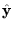from this distribution. There are many possible strategies for performing this selection with varying efficiencies and advantages. We consider and compare the following three approaches. One may select a random sample from, one may select the averageor one may compute thewith the highest probability.Sampling will often return a value which has a high probability however, it may sometimes return low values due to its inherent randomness. The average, i.e. the expectation, is a more consistent estimate but if the density is multimodal with more than one significant peak, thevalue returned might actually have low7.2 (as is the case in Figure 7.12). Thus, if we consistently wish to have a responsewith high probability, the best candidate is the highest peak in the marginal density, i.e. the arg max.Next: Expectation and Arg Max Up: CEM - A Maximum Previous: Conditional Constraints vs. Joint
Tony Jebara
1999-09-15